# Tensor Methods for Linear Pixel Manipulations¶

In 1994, Brian Smith introduced the first general method for computing arbitrary linear functions of image pixels in the JPEG transform domain (https://dl.acm.org/citation.cfm?id=192628). His methods not only allows for output pixels to be linear combinations of arbitrary input pixels, but also allows pixels to be reordered or selected according to linear rules. These notes develop his method by example starting with simple manipulations in the spatial domain to illustrate the mechanics of his method and building to arbitrary convolutions on JPEG compressed data.

This method is proposed to replace the convolutional operations in ResNet.

## Sample Data¶

A simple 16x16 RGB image is used as sample data. This image is chosen because it has a simple non-random pattern that can be understood at a glace, it is small enough that the high rank tensors used by some of the operations in the notebook can be used on it without running out of memory, and it will consist of 4 8x8 JPEG blocks which will be important later on. The details of the code below are not important, it simply loads the image and display it with a grayscale copy which will be used in the initial operations.

In :
%matplotlib inline

from cv2 import imread, cvtColor, COLOR_RGB2GRAY, COLOR_BGR2RGB, resize, INTER_NEAREST
from matplotlib import pyplot as plt
import numpy as np
import scipy.signal
import math
import os
from google.colab import drive

drive.mount('/content/gdrive')
impath = '/content/gdrive/My Drive/Colab Notebooks/Compressed Domain CNN/probe.png'

data = imread(impath)
data = cvtColor(data, COLOR_BGR2RGB)

img_gray = cvtColor(data, COLOR_RGB2GRAY)
data_gray = np.asarray(img_gray)

plt.figure(figsize=(10,10))
plt.subplot(1, 2, 1)
plt.grid(False)
plt.title('Color Sample Data')
plt.imshow(data)

plt.subplot(1, 2, 2)
plt.grid(False)
plt.title('Grayscale Sample Data')
plt.imshow(data_gray, cmap='gray');

Go to this URL in a browser: https://accounts.google.com/o/oauth2/auth?client_id=947318989803-6bn6qk8qdgf4n4g3pfee6491hc0brc4i.apps.googleusercontent.com&redirect_uri=urn%3Aietf%3Awg%3Aoauth%3A2.0%3Aoob&scope=email%20https%3A%2F%2Fwww.googleapis.com%2Fauth%2Fdocs.test%20https%3A%2F%2Fwww.googleapis.com%2Fauth%2Fdrive%20https%3A%2F%2Fwww.googleapis.com%2Fauth%2Fdrive.photos.readonly%20https%3A%2F%2Fwww.googleapis.com%2Fauth%2Fpeopleapi.readonly&response_type=code

Enter your authorization code:
··········
Mounted at /content/gdrive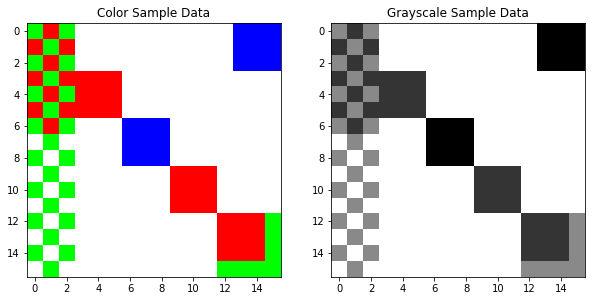## Multilinear Forms and Einstein Notation¶

The technique presented in these notes expresses pixel manipulations as multilinear forms, that is, arbitrary sums and products over different dimensions of sets of tensors. The accepted notation for these expressions is called Einstein Notation. This is used almost like an operation for applying the tensor operators to images, the major python libraries including numpy and pytorch all provide einsum functions that implement multilinear expressions using a derivitave of Einstein notation. Recall that in Einstein notation, any indices that are repated on the left hand side of an equation are summed over their range after element-wise mutiplication. Any indices still remaining on the right hand side of the equation are preserved (not summed out). While it is not required to re-write the preserved indices on the right hand side, it will always be done in these notes because it makes the form of the result easier to understand at a glance.

For example, assume that I have two vectors $x$ and $y$ of length 64 (rank 1 tensors). Then I could write

$$x_iy_i = z$$

to get the inner product $z$ of $x$ and $y$. This is equivilent to writing

$$\sum_{i=0}^{64} x_iy_i = z$$

Next, assume I have a matrix (rank 2 tensor) $Q$ of size (64, 32), then

$$Q^i_jx_i = q_j$$

is the matrix-vector product of $Q$ and $x$ Notice that the index $i$ is written in superscript, this is not a power. Notice also that the repeated index appears in superscript first and subscript second and the preserved index stays in position. This is not strictly required by Einstein notation but again it makes things clearer so it is followed strictly in these notes (the super and sub scripts do have meaning but it is not important for what we are doing here). The previous example is the same as writing

$$\sum_{i=0}^{64} Q_ix_i = q$$

Once nice thing about Einstein notation, besides that it is less verbose, is that it is immediatly clear that the result $q$ has size equal to the second index (32) and that it is a rank 1 tensor. Another neat trick of Einstein notation is that matrix multiplication becomes commutitave. This is because the standard notation for matrix multiplication makes an assumption about which index is being used based on the order of the matrices in the equation. Einstein notation makes no such assumption: the indices are always stated explicitly. In fact, using standard notation, the previous example is equivilent to

$$xQ = q$$

which is in the opposite order to what we have written. The convention of these notes is to always write the operator first, and the input second. So if I am convolving an image $I$ with a filter $C$, We would write

$$C^{i, j}_{u, v}I_{i, j} = I'_{u, v}$$

## Grayscale Smoothing¶

The grayscale version of the image is a (16,16) matrix (rank 2 tensor). This is the simplest form we can work with, so the first example is a gaussian smoothing filter on this grayscale image. For this, a rank 4 tensor is needed.

Think of this tensor as indexing each dimension in each image separately. For example, let's call the input image axes (i, j). Since the output is the smoothed version of the input, it will also be a grayscale image, so call it's axes (u, v). Then the tensor operator will be indexed as (i, j, u, v) where the entry in the operator at (i, j, u, v) gives the coefficent for pixel (i, j) in the input and (u, v) in the output. The final output is then obtained by summing the contributions to the same pixel (u, v). Unfortunatly, this is impossible to visualize since the tensor operator is four dimensional, so the intuition and code will have to suffice.

Make sure that you understand this section and the code before continuing because it only gets more complicated.

In :
def smoothing_tensor(shape):
op = np.zeros((shape, shape, shape, shape))
for i in range(0, shape):
for j in range(0, shape):
for u in range(0, shape):
for v in range(0, shape):
if i == u and j == v:
op[i, j, u, v] = 0.5
elif i == u and (j == (v - 1) or j == (v + 1)):
op[i, j, u, v] = 0.125
elif (i == (u - 1) or i == (u + 1)) and j == v:
op[i, j, u, v] = 0.125

return op

smooth_op = smoothing_tensor(data_gray.shape)
print(smooth_op.shape)

res = np.einsum('ijuv,ij->uv', smooth_op, data_gray)
print(res.shape)

plt.figure()
plt.grid(False)
plt.imshow(res / 255, cmap='gray');

(16, 16, 16, 16)
(16, 16)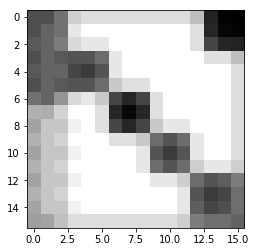## Color to Grayscale¶

As a fun example that is still sufficiently simple, we can define a tensor that converts color images to grayscale using the standard empirical formula

$$i = 0.299r + 0.587b + 0.114g$$

this is illustrative of the power of these tensor operators: this is a somewhat complex operation expressed concisely.

The operator for this is a rank 5 tensor with indices (i, j, c, u, v). i and j index the pixel in the input image, c indices the color channel (there are 3 of them), and (u, v) indexes the grayscale pixel in the output image. In Einstein notation, the formula is

$$G^{i, j, c}_{u, v} I_{i, j, c} = I'_{u ,v}$$

indicating that after multiplying, we sum overall (i, j, c).

Notice that this tensor is of size (16, 16, 3, 16, 16): already the tensors are getting quite big, although most of their entries are 0s.

In the output of the code below, the grayscale image as converted by the OpenCV library (known good implementation) is compared with they grayscale image created by our tensor operator. They are of course identical.

In :
def grayscale_tensor(shape):
op = np.zeros((shape, shape, shape, shape, shape))
for i in range(0, shape):
for j in range(0, shape):
for u in range(0, shape):
for v in range(0, shape):
if i == u and v == j:
op[i, j, 0, u, v] = 0.299
op[i, j, 1, u, v] = 0.587
op[i, j, 2, u, v] = 0.114

return op

grayscale_op = grayscale_tensor(data.shape)
print(grayscale_op.shape)

res_gray = np.einsum('ijcuv,ijc->uv', grayscale_op, data)
print(res_gray.shape)

plt.figure(figsize=(10,10))
plt.subplot(1, 2, 1)
plt.grid(False)
plt.imshow(res_gray, cmap='gray')
plt.subplot(1, 2, 2)
plt.grid(False)
plt.imshow(data_gray, cmap='gray');

(16, 16, 3, 16, 16)
(16, 16)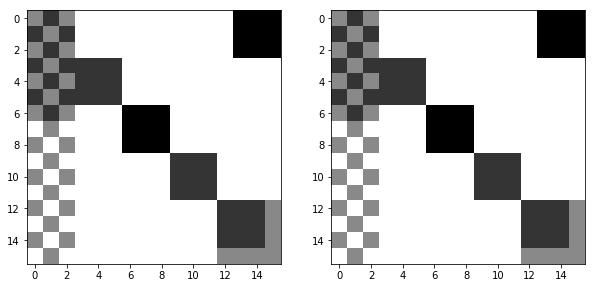## More Dimensions¶

Next lets move to a higher dimensional transform and define the same guassian smoothing operation on the color image. An interesting result of this is that it is actually the same tensor that was used for the grayscale smoothing. In effect, it is actually the grayscale operation applied separately to each channel. Consider the operation in Einstein notation

$$S^{i, j}_{u, v} I_{i, j, c} = I'_{u, v, c}$$

Since $c$ is not repeated on the left hand side, there is no need to sum over it, and the tensor does not need an extra dimension.

In :
def smoothing_tensor_color(shape):
op = np.zeros((shape, shape, shape, shape))
for i in range(0, shape):
for j in range(0, shape):
for u in range(0, shape):
for v in range(0, shape):
if i == u and j == v:
op[i, j, u, v] = 0.5
elif i == u and (j == (v - 1) or j == (v + 1)):
op[i, j, u, v] = 0.125
elif (i == (u - 1) or i == (u + 1)) and j == v:
op[i, j, u, v] = 0.125

return op

smooth_op_color = smoothing_tensor_color(data.shape)
print(smooth_op_color.shape)

res_col = np.einsum('ijuv,ijc->uvc', smooth_op_color, data)
print(res_col.shape)

plt.figure()
plt.grid(False)
plt.imshow(res_col / 255, cmap='gray');

(16, 16, 16, 16)
(16, 16, 3)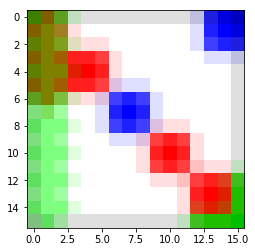## Resampling¶

So far we have considered only transformations that preserve image size, but we can also create tensors that do image resizing/resampling. Consider the tensor of shape (16, 16, 8, 8) where an index $(i, j, u, v)$ is 1 if $i = 2u \land j = 2v$ and 0 otherwise. This tensor performs nearest neighbor downsampling by half. This is demonstrated in the code below and the same tensor can be used to resize grayscale and color images. The result on both grayscale and color is shown along with the resampling result using the OpenCV library, which is identical.

In :
def half_size_tensor(shape):
op = np.zeros((shape, shape, shape // 2, shape // 2))
for i in range(0, shape):
for j in range(0, shape):
for u in range(0, shape // 2):
for v in range(0, shape // 2):
if i == 2*u and j == 2*v:
op[i, j, u, v] = 1

return op

half_size_op = half_size_tensor(data.shape)
print(half_size_op.shape)

res = np.einsum('ijuv,ij->uv', half_size_op, data_gray)
print(res.shape)

res_col = np.einsum('ijuv,ijc->uvc', half_size_op, data)
print(res_col.shape)

res_true = resize(data, (8, 8), interpolation=INTER_NEAREST)

plt.figure(figsize=(20, 10))

plt.subplot(1, 3, 1)
plt.grid(False)
plt.imshow(res / 255, cmap='gray')
plt.title('Grayscale NN Tensor')

plt.subplot(1, 3, 2)
plt.grid(False)
plt.imshow(res_col / 255)
plt.title('Color NN Tensor')

plt.subplot(1, 3, 3)
plt.grid(False)
plt.imshow(res_true / 255, cmap='gray')
plt.title('Color NN from Library');

(16, 16, 8, 8)
(8, 8)
(8, 8, 3)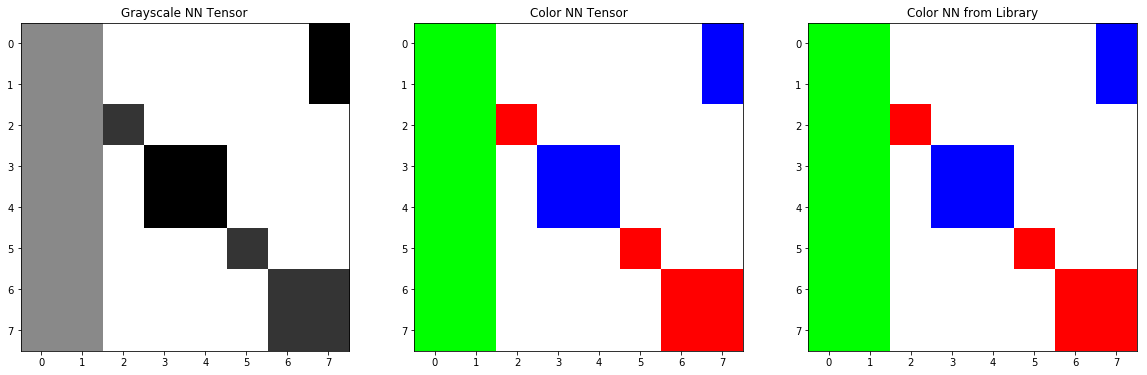Of course this works for upsizing as well, which is shown below.

In :
def double_size_tensor(shape):
op = np.zeros((shape, shape, shape * 2, shape * 2))
for i in range(0, shape):
for j in range(0, shape):
for u in range(0, shape * 2):
for v in range(0, shape * 2):
if i == u // 2 and j == v // 2:
op[i, j, u, v] = 1

return op

double_size_op = double_size_tensor(data.shape)
print(double_size_op.shape)

res = np.einsum('ijuv,ij->uv', double_size_op, data_gray)
print(res.shape)

res_col = np.einsum('ijuv,ijc->uvc', double_size_op, data)
print(res_col.shape)

res_true = resize(data, (32, 32), interpolation=INTER_NEAREST)

plt.figure(figsize=(20, 10))

plt.subplot(1, 3, 1)
plt.grid(False)
plt.imshow(res / 255, cmap='gray')
plt.title('Grayscale NN Tensor')

plt.subplot(1, 3, 2)
plt.grid(False)
plt.imshow(res_col / 255)
plt.title('Color NN Tensor')

plt.subplot(1, 3, 3)
plt.grid(False)
plt.imshow(res_true / 255, cmap='gray')
plt.title('Color NN from Library');

(16, 16, 32, 32)
(32, 32)
(32, 32, 3)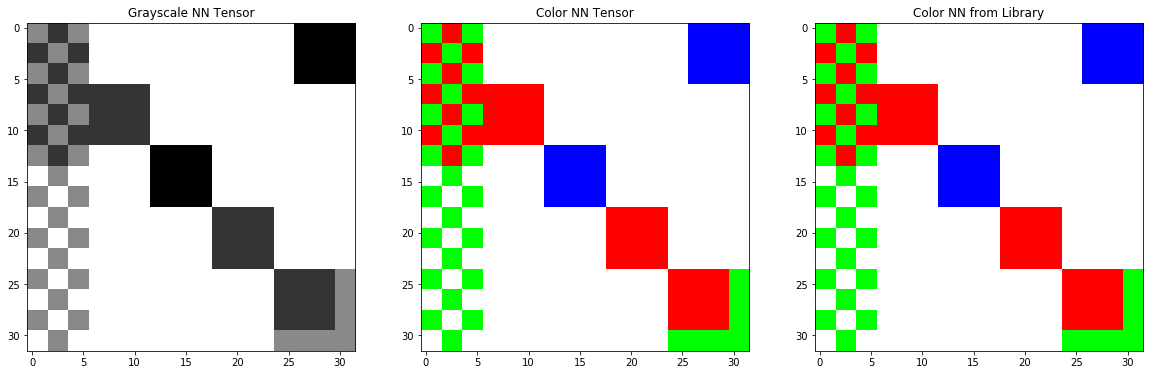Other resizing methods work just as well, nearest neighbors resizing was used for illustrative purposes only because of its simplicity.

## Exploding Convolutions¶

Let's move on the performing arbitrary convolutions. The code below computes a random $5 \times 5$ convolution and creates a tensor operator out of it, then applies it to our example image including padding for edge effects. I call this algorithm "exploding" the convolution since the small $5 \times 5$ matrix is expanded to be a $(w, h, w ,h)$ rank 4 tensor. In Einstein notation, this is computed in the same way as the smoothing operation

$$C^{i, j}_{u, v} I_{i, j} = I'_{u, v}$$

In the code below, the random convolution is first computed using the scipy library (a known good implementation), then computed using the tensor operator. The result is nearly identical, and this is shown numerically in an image of the difference between the two results. The sum of this difference image is also printed to quantify the error over the entire image. The numerical results show that the results are the same to within a floating point error.

In :
def randconv(size):
conv = np.random.normal(0, np.sqrt(size), (size, size))
conv /= np.linalg.norm(conv)
return conv
# conv = np.array([[ 0.03323893, -0.26312419,  0.28235156],
#                  [-0.29062169, -0.36743651, -0.54203082],
#                  [-0.31836532, -0.30061654,  0.38071469]])

conv = randconv(5)
res_conv = scipy.signal.convolve2d(data_gray, conv, mode='same')
plt.figure(figsize=(20, 10))
plt.subplot(1, 3, 1)
plt.grid(False)
plt.title('Convolution')
plt.imshow(res_conv / 255, cmap='gray')

def conv_to_tensor(shape, conv):
size = (math.floor(conv.shape / 2), math.floor(conv.shape / 2))
shape = (shape + size * 2, shape + size * 2)

op = np.zeros((shape, shape, shape, shape))
for i in range(0, shape):
for j in range(0, shape):
for u in range(0, shape):
for v in range(0, shape):
hrange = (u - size,
u + size)
vrange = (v - size,
v + size)

if hrange <= i <= hrange and vrange <= j <= vrange:
x = u - i + size
y = v - j + size
op[i, j, u, v] = conv[x, y]
return op

def apply_conv_tensor(op, im):
pad_size = (math.floor(conv.shape / 2), math.floor(conv.shape / 2))

padded = np.zeros((im.shape + pad_size * 2, im.shape + pad_size * 2))
padded[pad_size:im.shape+pad_size, pad_size:im.shape+pad_size] = im
res_padded = np.einsum('ijuv,ij->uv', op, padded)
return res_padded[pad_size:im.shape+pad_size, pad_size:im.shape+pad_size]

conv_op = conv_to_tensor(data_gray.shape, conv)
res_tconv = apply_conv_tensor(conv_op, data_gray)
plt.subplot(1, 3, 2)
plt.grid(False)
plt.title('Tensor Operator')
plt.imshow(res_tconv / 255, cmap='gray')

res_diff = np.abs(np.subtract(res_tconv, res_conv))
print('Error: {}'.format(np.sum(res_diff)))

plt.subplot(1, 3, 3)
plt.grid(False)
plt.title('Error')
plt.imshow(res_diff, cmap='gray');

Error: 6.4996896753655165e-12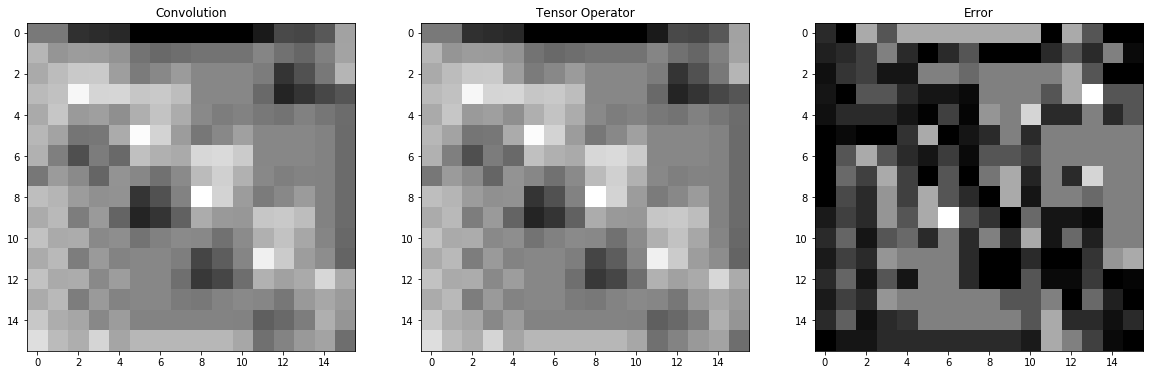Of course in CNNs, convolutions are not applied to single channels, they are instead applied accross channels yielding a single channel result per convolution (speaking in approximate terms, different models have different takes on this). The following code implements this kind of convolution. Although the same sample data is used as input (this time the color version), imagine that it is an input from a hidden layer which had three convolutions. The code below implements a single convolution on this volume. In Einstien notation, the operation is

$$C^{i, j, d}_{u, v} I_{i, j, d} = I'_{u, v}$$

In a layer with several convolutions, multiple $I'$ would be stacked to produce the activation from each convolution before non-linearity is applied and the result is passed to the next layer. Convolving volumes in this way is not directly supported by the scipy implementation we are comparing against, but a simple implementation is provided that computes the convolution separately along each channel and then sums the channels.

As in the last example, the output is first computed using the scipy library to have a known good result, then the result is computed using the tensor operator, created using the same exploding convolution method. The difference between the known good result and the tensor operator result is computed and again shown to be within a floating point error.

In :
def apply_conv_volume(conv, im):
res_conv = np.zeros(im.shape)
for d in range(im.shape):
res_conv[:, :, d] = scipy.signal.convolve2d(im[:, :, d], conv, mode='same')

return np.sum(res_conv, axis=2)

res_conv_volume = apply_conv_volume(conv, data)

plt.figure(figsize=(20, 10))
plt.subplot(1, 3, 1)
plt.grid(False)
plt.title('Convolution')
plt.imshow(res_conv_volume / 255, cmap='gray')

def conv_to_tensor_volume(shape, conv):
size = (math.floor(conv.shape / 2), math.floor(conv.shape / 2))
shape = (shape + size * 2, shape + size * 2, shape)

op = np.zeros((shape, shape, shape, shape, shape))
for i in range(0, shape):
for j in range(0, shape):
for u in range(0, shape):
for v in range(0, shape):
hrange = (u - size,
u + size)
vrange = (v - size,
v + size)

if hrange <= i <= hrange and vrange <= j <= vrange:
x = u - i + size
y = v - j + size

op[i, j, :, u, v] = conv[x, y]
return op

def apply_conv_tensor_volume(op, im):
pad_size = (math.floor(conv.shape / 2), math.floor(conv.shape / 2))

padded = np.zeros((im.shape + pad_size * 2, im.shape + pad_size * 2, im.shape))
padded[pad_size:im.shape+pad_size, pad_size:im.shape+pad_size] = im
res_padded = np.einsum('ijduv,ijd->uv', op, padded)
return res_padded[pad_size:im.shape+pad_size, pad_size:im.shape+pad_size]

conv_op_volume = conv_to_tensor_volume(data.shape, conv)
res_tconv_volume = apply_conv_tensor_volume(conv_op_volume, data)
plt.subplot(1, 3, 2)
plt.grid(False)
plt.title('Tensor Operator')
plt.imshow(res_tconv_volume / 255, cmap='gray')

res_diff_volume = np.abs(np.subtract(res_tconv_volume, res_conv_volume))
print('Error: {}'.format(np.sum(res_diff_volume)))

plt.subplot(1, 3, 3)
plt.grid(False)
plt.title('Error')
plt.imshow(res_diff_volume, cmap='gray');

Error: 4.532729747097619e-11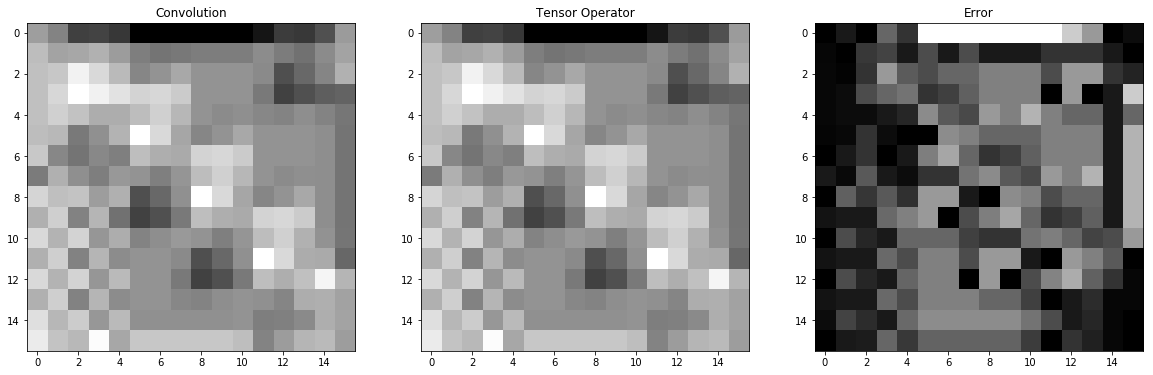And as a last result, resizing can be performed at the same time using strided convolutions. This is an important feature of ResNet which replaces almost all pooling with strided convolutions. Strided convolutions are not directly supported by scipy, but a simple implementation that uses the full convolution (stride=1) then takes every stride pixels from the output is provided. Again the result is within a floating point error.

In :
def apply_conv_volume_strided(conv, im, stride):
return apply_conv_volume(conv, im)[::stride,::stride]

res_conv_volume = apply_conv_volume_strided(conv, data, (2, 2))

plt.figure(figsize=(20, 10))
plt.subplot(1, 3, 1)
plt.grid(False)
plt.title('Convolution')
plt.imshow(res_conv_volume / 255, cmap='gray')

def conv_to_tensor_volume_strided(shape, conv, stride):
size = (math.floor(conv.shape / 2), math.floor(conv.shape / 2))
shape = (shape + size * 2, shape + size * 2, shape)

op = np.zeros((shape, shape, shape, shape // stride, shape // stride))
for i in range(0, shape):
for j in range(0, shape):
for u in range(0, shape // stride):
for v in range(0, shape // stride):
hrange = (stride * u - size,
stride * u + size)
vrange = (stride * v - size,
stride * v + size)

if hrange <= i <= hrange and vrange <= j <= vrange:
x = stride * u - i + size
y = stride * v - j + size

op[i, j, :, u, v] = conv[x, y]
return op

def apply_conv_tensor_volume_strided(op, im, stride):
pad_size = (math.floor(conv.shape / 2), math.floor(conv.shape / 2))

padded = np.zeros((im.shape + pad_size * 2, im.shape + pad_size * 2, im.shape))
padded[pad_size:im.shape+pad_size, pad_size:im.shape+pad_size] = im

res_padded = np.einsum('ijduv,ijd->uv', op, padded)
return res_padded[(pad_size // stride):(im.shape+pad_size) // stride, (pad_size//stride):(im.shape+pad_size)//stride]

conv_op_volume = conv_to_tensor_volume_strided(data.shape, conv, (2, 2))
res_tconv_volume = apply_conv_tensor_volume_strided(conv_op_volume, data, (2, 2))
plt.subplot(1, 3, 2)
plt.grid(False)
plt.title('Tensor Operator')
plt.imshow(res_tconv_volume / 255, cmap='gray')

res_diff_volume = np.abs(np.subtract(res_tconv_volume, res_conv_volume))
print('Error: {}'.format(np.sum(res_diff_volume)))

plt.subplot(1, 3, 3)
plt.grid(False)
plt.title('Error')
plt.imshow(res_diff_volume, cmap='gray');

Error: 1.1784351272581262e-11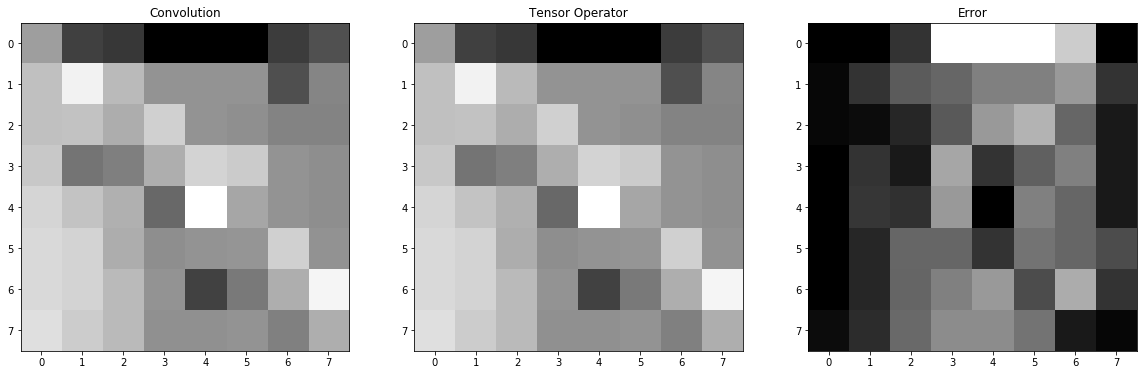## JPEG as a Tensor¶

So far there has been no motivation for why we would bother with this at all. In this section, the tensor operator for JPEG compression is developed. It may be surprising at first, but there are only two steps in jpeg compression that are non-linear and therefore cannot be expressed with tensor operators. To review, the (approximate) steps of the JPEG compression algorithm are as follows:

1. Break the image into $8 \times 8$ blocks this is linear
2. Center the blocks (scale it to $[-127, 127]$) this is linear (and skipped in the code below. In a CNN a batch norm layer would have taken care of this)
3. Compute the $8 \times 8$ discrete cosine transform coefficients of each block, this is linear
4. Reorder the pixels in "zig-zag", or morton, order, this is linear
5. Scale the reordered coefficients by a quantization matrix this is linear
6. Quantize the coefficients by rounding to the nearest integer, this is NOT linear (and it can be skipped in most cases)
7. Entropy code the blocks, this is NOT linear and cant be skipped

The last step of entropy coding will need to be undone before any JPEG data can be processed. The only other nonlinear step, the quantization step, is simply skipped by most compressed domain processing algorithms, since it is also skipped by all decompressors. This is the lossy step in JPEG.

### Blockifying and Reordering Pixels is Linear?¶

Yes they are, this is the major contribution of Brian Smith's tensor method and is what allows for arbitrary linear functions to be computed. Let's go back the the grayscale image, and remember that it was indexed as (i, j), its output was indexed as (u, v) and therefore a tensor operation on it would be indexed as (i, j, u, v) where each entry gives the coefficient for input pixel (i, j)s contribution to output pixel (u, v). So what if the tensor operation had entry (5, 5, 2, 2) as 1? Then it would mean move pixel (5, 5) in the input image to (2, 2) in the output image (assiming there are no other contributions to pixel (2. 2)). This is how the zig-zag reordering is implemented as a linear operation.

Splitting the image into blocks is implemented in a similar way, though it is a little more complex. Take a rank 6 tensor $B$ and index it as $(s_x, s_y, x, y, i, j)$ where $(s_x, s_y)$ is the position of a pixel in the input image, $(x, y)$ is the spatial position of a block (for our $16 \times 16$ example the valid values for $x$ and $y$ would be 0 or 1), and $(i, j)$ is the offset into the block. For each input pixel $(s_x, s_y)$, set the $(s_x, s_y, x, y, i, j)$ entry of $B$ to 1 if $(s_x, s_y)$ should be in block $(x, y)$ at offset $(i, j)$ and 0 otherwise. Then the following operation

$$B^{s_x, s_y}_{x, y, i, j}I_{s_x, s_y} = L_{x, y, i, j}$$

breaks the image $I$ into $(x, y)$ blocks each of size $(i, j)$. This is step 1 in the JPEG algorithm.

### Other JPEG Steps¶

The other steps of the JPEG algorithm are a little more straightfoward.

The DCT is performed on each block, it can be represented with a rank 4 (8, 8 , 8, 8) tensor $D$. For a block entry $(i, j)$, the $(i, j, \alpha, \beta)$ entry contains the coefficient for spatial position $(i, j)$ and frequency $(\alpha,\beta)$. The form of the coefficients follows directly from the DCT (see "Understanding the DCT" notes). It is implemented as

$$D^{i, j}_{\alpha, \beta} L_{x, y, i, j} = \Phi_{x, y, \alpha, \beta}$$

Reodering the pixels in zig-zag order was already discussed, but we also take this opporunity to form was is called a "sparse code" which is just taking the $8 \times 8$ blocks and rewriting them after the reordering as 64 element vectors. This order is hard-coded in the code below to make the implementation simpler. Let the rank 3 tensor $Z$ be of indexed as $(\alpha, \beta, \gamma)$ where the entry is 1 if spatial frequency $(\alpha, \beta)$ belongs at location $\gamma$ in zig-zag ordering, and 0 otherwise. Then

$$Z^{\alpha, \beta}_\gamma \Phi_{x, y, \alpha, \beta} = C_{x, y, \gamma}$$

implements the reodering

The final step is scaling the coefficients by the quantiazation matrix. Let $Q$ be a rank 2 tensor indexed as $(\gamma, k)$ where the entry gives the quantization coefficient for entry $\gamma$ in the sparse code. Then

$$Q^\gamma_k C_{x, y, \gamma} = F_{x, y, k}$$

Where the final tensor $F$ gives each of the sparse codes in its block position $(x, y)$. The entries of this tensor can be rounded and then entropy coded to store on disk as a JPEG file.

### Putting It Together¶

Since all of these separate tensors are linear operations, they can all be combined into a single tensor. This tensor would give the coefficient for each pixel and map it directly to a sparse code. Precomputing this tensor allows the JPEG operation to be performed quickly. The rank 5 tensor $J$ such that

$$B^{s_x, s_y}_{x, y, i, j}D^{i, j}_{\alpha, \beta}Z^{\alpha, \beta}_\gamma Q^\gamma_k = J^{s_x, s_y}_{x, y, k}$$

implements JPEG compression and is applied as

$$J^{s_x, s_y}_{x, y, k} I_{s_x, s_y} = F_{x, y, k}$$

JPEG decompression can of course also be implemented as a tensor $\hat{J}$ and is derived in the same way as JPEG compression but using the inverse operations. Now, when performing an arbitrary convolution $C$ on a JPEG compressed image $F$, given as sparse codes, we can perform the following operations:

1. $\hat{J}^{x, y, k}_{s_x, s_y}F_{x, y, k} = I_{s_x, s_y}$ to get the decompressed image $I$
2. $C^{s_x, s_y}_{s'_x, s'_y}I^{s'_x, s'_y}_{s_x, s_y} = I'_{s'_x, s'_y}$ to apply the convolution $C$ to $I$ using a tensor operator
3. $J^{s'_x, s'_y}_{x', y', k'} I'_{s'_x, s'_y} = F'_{x', y', k'}$ to get the compressed result $F'$ as sparse codes

or we can do even better and compute

$$\hat{J}^{x, y, k}_{s_x, s_y}C^{s_x, s_y}_{s'_x, s'_y}J^{s'_x, s'_y}_{x', y', k'} = \Xi^{x, y, k}_{x', y', k'}$$

which follows from the above steps by:

$$\hat{J}^{x, y, k}_{s_x, s_y}F_{x, y, k} = I_{s_x, s_y} \\ C^{s_x, s_y}_{s'_x, s'_y}I^{s'_x, s'_y}_{s_x, s_y} = C^{s_x, s_y}_{s'_x, s'_y}\hat{J}^{x, y, k}_{s_x, s_y}F_{x, y, k} = I'_{s'_x, s'_y} \\ J^{s'_x, s'_y}_{x', y', k'} I'_{s'_x, s'_y} = J^{s'_x, s'_y}_{x', y', k'} C^{s_x, s_y}_{s'_x, s'_y}\hat{J}^{x, y, k}_{s_x, s_y}F_{x, y, k} = F'_{x', y', k'} \\ \left[J^{s'_x, s'_y}_{x', y', k'} C^{s_x, s_y}_{s'_x, s'_y}\hat{J}^{x, y, k}_{s_x, s_y}\right]F_{x, y, k} = F'_{x', y', k'} \\ \Xi^{x, y, k}_{x', y', k'}F_{x, y, k} = F'_{x', y', k'}$$

This new tensor computes the operation $C$ directly on the sparse codes without doing any decompression. In effect, what this procedure does is to plug in all the parts of the JPEG compression, decompresson, and coefficients from the convolution, then multiply them all out into a single coefficient per sparse code entry per block. This is one of the the results that Smith's tensor method was designed for. Now any operation that can be expressed as a tensor can be performed on JPEG compressed images in a highly efficient manner.

Smith went on to describe an approximation method based on this work called a condensation which increases the sparsity of both the original JPEG and the tensor operators but at the cost of decreased accuracy. This method is not considered here.

An important thing to note about $\Xi$ is that applying it to the compressed image is mathematically equivilent to applying C on the decompressed image and compressing the result. There is no loss of accuracy or approximation, the only differences will be from floating point errors.

### Code¶

Let's start by implementing the parts of the JPEG compression tensor. Each function in the code below implements one part of the JPEG algorithm. Their results are then combined to create the JPEG compression tensor $J$.

• The functions A() and D() implement the $8 \times 8$ DCT.
• The function Z() imlements the zig-zag reordering
• The function S() implements scaling by a quantization matrix (the matrix comes from libjpeg average quality)
• The function B() breaks the image into $8 \times 8$ blocks

Because of the number of arguments that need to be combined and the high rank of the tensors, this operation can take some time to complete, but keep in mind this tensor can be precomputed offline and stored, it need not be computed every time it is needed.

In :
def A(alpha):
if alpha == 0:
return 1.0 / math.sqrt(2)
else:
return 1

def D():
D_t = np.zeros((8, 8, 8, 8))

for i in range(8):
for j in range(8):
for alpha in range(8):
for beta in range(8):
scale_a = A(alpha)
scale_b = A(beta)

coeff_x = math.cos(((2 * i + 1) * alpha * math.pi) / 16)
coeff_y = math.cos(((2 * j + 1) * beta * math.pi) / 16)

D_t[i, j, alpha, beta] = 0.25 * scale_a * scale_b * coeff_x * coeff_y
return D_t

def Z():
z = np.array([[ 0,  1,  5,  6, 14, 15, 27, 28],
[ 2,  4,  7, 13, 16, 26, 29, 42],
[ 3,  8, 12, 17, 25, 30, 41, 43],
[ 9, 11, 18, 24, 31, 40, 44, 53],
[10, 19, 23, 32, 39, 45, 52, 54],
[20, 22, 33, 38, 46, 51, 55, 60],
[21, 34, 37, 47, 50, 56, 59, 61],
[35, 36, 48, 49, 57, 58, 62, 63]], dtype=float)

Z_t = np.zeros((8, 8, 64))
for alpha in range(8):
for beta in range(8):
for gamma in range(64):
if z[alpha, beta] == gamma:
Z_t[alpha, beta, gamma] = 1

return Z_t

def S():
q = np.array([ 8, 16, 16, 19, 16, 19, 22, 22, 22, 22, 22, 22, 26, 24, 26, 27,
27, 27, 26, 26, 26, 26, 27, 27, 27, 29, 29, 29, 34, 34, 34, 29,
29, 29, 27, 27, 29, 29, 32, 32, 34, 34, 37, 38, 37, 35, 35, 34,
35, 38, 38, 40, 40, 40, 48, 48, 46, 46, 56, 56, 58, 69, 69, 83], dtype=float)

S_t = np.zeros((64, 64))
for gamma in range(64):
for k in range(64):
if gamma == k:
S_t[gamma, k] = 1.0 / q[k]

return S_t

def B(shape, block_size):
blocks_shape = (shape // block_size, shape // block_size)
B_t = np.zeros((shape, shape, blocks_shape, blocks_shape, block_size, block_size))

for s_x in range(shape):
for s_y in range(shape):
for x in range(blocks_shape):
for y in range(blocks_shape):
for i in range(block_size):
for j in range(block_size):
if x * block_size + i == s_x and y * block_size + j == s_y:
B_t[s_x, s_y, x, y, i, j] = 1.0
return B_t

J = np.einsum('srxyij,ijab,abg,gk->srxyk', B(data.shape, (8, 8)), D(), Z(), S())
print(J.shape)

(16, 16, 2, 2, 64)


$\hat{J}$ can by computed from the above functions, in fact only the only change is that the quantization matrix needs to be inverted

In :
def S_i():
q = np.array([ 8, 16, 16, 19, 16, 19, 22, 22, 22, 22, 22, 22, 26, 24, 26, 27,
27, 27, 26, 26, 26, 26, 27, 27, 27, 29, 29, 29, 34, 34, 34, 29,
29, 29, 27, 27, 29, 29, 32, 32, 34, 34, 37, 38, 37, 35, 35, 34,
35, 38, 38, 40, 40, 40, 48, 48, 46, 46, 56, 56, 58, 69, 69, 83], dtype=float)

S_t = np.zeros((64, 64))
for gamma in range(64):
for k in range(64):
if gamma == k:
S_t[gamma, k] = q[k]

return S_t

J_i = np.einsum('srxyij,ijab,abg,gk->xyksr', B(data.shape, (8, 8)), D(), Z(), S_i())
print(J_i.shape)

(2, 2, 64, 16, 16)


It's a little hard to visualize the JPEG after transforming, but we can play with the individual parts of the transform to get an idea for how it works. In the next section, the example image is blockified and then the DCT coefficients are computed. In other words, we first compute

$$B^{s_x,s_y}_{x,y,i,j}I_{s_x,s_y} = L_{x,y,i,j}$$

which is shown in the first image, then we compute

$$D^{i, j}_{\alpha,\beta}L_{x, y, i, j} = \Phi_{x,y,\alpha, \beta}$$

which is shown in the second image

In :
def show_blocks(block_data, title):
blocks_x = block_data.shape
blocks_y = block_data.shape

fig = plt.figure(figsize=(5, 5))
fig.suptitle(title)
for i in range(blocks_x):
for j in range(blocks_y):
plt.subplot(blocks_x, blocks_y, blocks_y * i + j + 1)
plt.grid(False)
plt.imshow(block_data[i, j], cmap='gray');

block_data = np.einsum('srxyij,sr->xyij',B(data.shape, (8, 8)), data_gray)
dct_blocks = np.einsum('ijab,xyij->xyab', D(), block_data)

show_blocks(block_data, 'Blockified Image')
show_blocks(dct_blocks, 'DCT Blocks')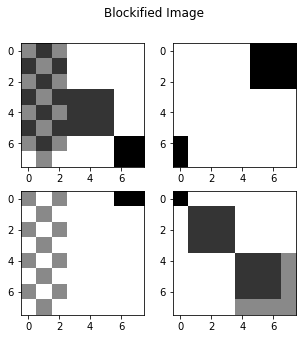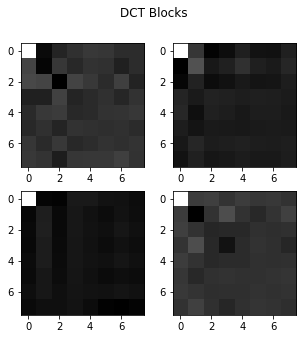Next let's apply the JPEG tensor. We apply this formula

$$J^{s_x, s_y}_{x, y, k}I_{s_x, s_y}\hat{J}^{x, y, k}_{s_x, s_y} = I_{s_x, s_y}$$

and show that the result is within a foating point error. Keep in mind that because we have not rounded the coefficients, this is a lossless "compression".

In :
jpeg_data = np.einsum('srxyk,sr->xyk', J, data_gray)
data_copy = np.einsum('xyksr,xyk->sr', J_i, jpeg_data)

res_diff = np.abs(np.subtract(data_gray, data_copy))
print('Error: {}'.format(np.sum(res_diff)))

plt.figure(figsize=(20, 10))
plt.subplot(1, 3, 1)
plt.grid(False)
plt.title('Original Image')
plt.imshow(data_gray, cmap='gray');

plt.subplot(1, 3, 2)
plt.grid(False)
plt.title('After Compression and Decompression')
plt.imshow(data_copy, cmap='gray');

plt.subplot(1, 3, 3)
plt.grid(False)
plt.title('Error')
plt.imshow(res_diff, cmap='gray');

Error: 3.268496584496461e-11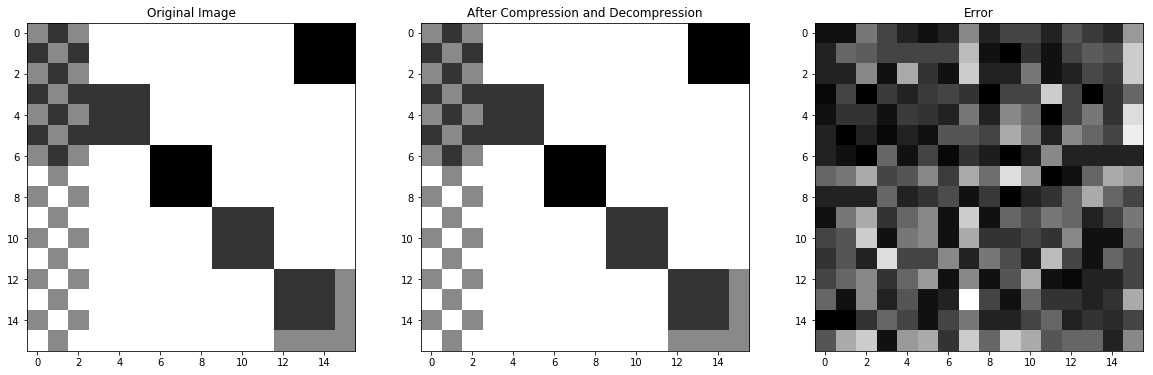Finally, let's apply the simple convolution from the "Exploding Convolutions" section to the compressed image and show that it matches the decompressed one. For simplicity I skip the zero padding that was used in the convolution examples.

In :
def conv_to_tensor_no_padding(shape, conv):
size = (math.floor(conv.shape / 2), math.floor(conv.shape / 2))

op = np.zeros((shape, shape, shape, shape))
for i in range(0, shape):
for j in range(0, shape):
for u in range(0, shape):
for v in range(0, shape):
hrange = (u - size,
u + size)
vrange = (v - size,
v + size)

if hrange <= i <= hrange and vrange <= j <= vrange:
x = u - i + size
y = v - j + size
op[i, j, u, v] = conv[x, y]
return op

conv_op = conv_to_tensor_no_padding(data_gray.shape, conv)
conv_op_compressed = np.einsum('xyksr,srpq,pqabc->xykabc', J_i, conv_op, J)

res_spatial = np.einsum('srpq,sr->pq', conv_op, data_gray)
res_compres = np.einsum('xykabc,xyk->abc', conv_op_compressed, jpeg_data)

res_decomp = np.einsum('xyksr,xyk->sr', J_i, res_compres)

res_diff = np.abs(np.subtract(res_decomp, res_spatial))
print('Error: {}'.format(np.sum(res_diff)))

plt.figure(figsize=(20, 10))
plt.subplot(1, 3, 1)
plt.grid(False)
plt.title('Applying Conv Decompressed')
plt.imshow(res_spatial, cmap='gray');

plt.subplot(1, 3, 2)
plt.grid(False)
plt.title('Applying Conv Compressed')
plt.imshow(res_decomp, cmap='gray');

plt.subplot(1, 3, 3)
plt.grid(False)
plt.title('Error')
plt.imshow(res_diff, cmap='gray');

Error: 8.714229338124824e-11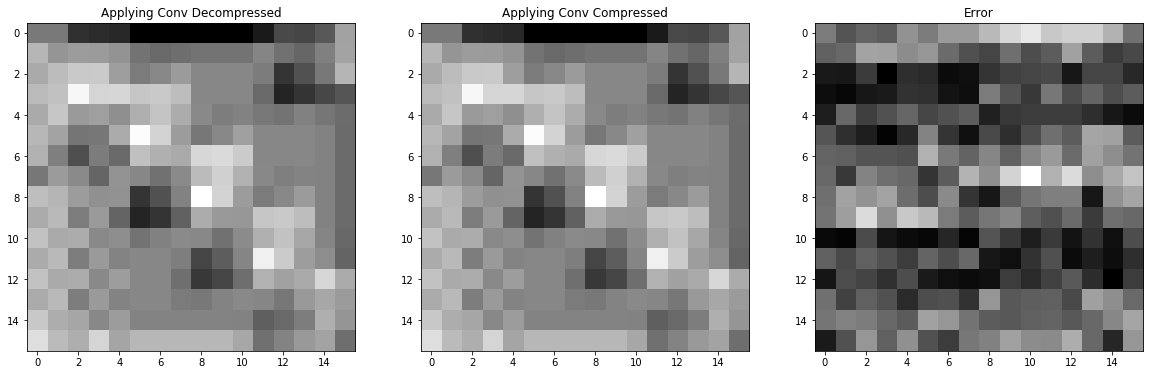This final example is what these notes have been building to. The convolution is applied to the JPEG data directly, without decompressing it, and is identical to the result of applying the convolution to the decompressed image, save for floating point error. Additional pieces like includding zero padding or convolving over volumes, which were demonstrated earlier, follow directly from this example.

## A Note On Efficient Storage¶

One thing that may have become apparent is that the tensor representation for even simple convolutions is extremely large, proportional to the size of the image. In the last example, the convolution was a rank 6 tensor of shape $(2,2,64,2,2,64)$, or 65536 elements. That is certainly a lot more storage than the original $5 \times 5$ convoltution with 25 elements.

The canonical solution to this problem is to use a sparse tensor to store the results. Examining the last tensor reveals that it is highly sparse:

In :
total_size = np.prod(conv_op_compressed.shape)
num_nonzero = np.count_nonzero(conv_op)

print('Nonzero Elements: {}, Total Size: {}, Density: {}'.format(num_nonzero, total_size, num_nonzero / total_size))

Nonzero Elements: 5476, Total Size: 65536, Density: 0.08355712890625


where 8% density indicates that only 8% of the elements are set. This is certainly a workable solution, but in my opinion it is still not ideal. Considering that only 25 elements were required for the original convolution, those 5476 elements in the tensor operator should be a repeated pattern of the original 25. Reducing the tensor operator to this minimal representation is ongoing work.

© 2018 Max Ehrlich